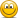# CourseErrors Found in 2021/2022 Study Materials P2.T5. Market Risk

#### Nicole Seaman

##### Director of FRM Operations
Staff member
Please use this new thread to let David and I know about any errors, missing/broken links, etc. that you find in the 2021/2022 materials that are published in the study planner under P1.T4. Valuation & Risk Models. This will keep our forum much more organized. We appreciate your cooperation!PLEASE NOTE: Our Practice Question sets already have links to their specific forum threads where you can post about any errors that you find. This thread is for any other materials (notes, spreadsheets, videos, etc.) where you might find errors.

Information needed for us to correct errors:

• Page number
• Error

Last edited:

#### kchristo

##### Member
In T5-R1-P2-Dowd, under "Define coherent risk measures.", the definition of positive homogeniety refers to X and Y in the image but only X in the text. (P10)

#### poojanmehta1

##### New Member
@Nicole Seaman - Am looking at pdf notes of Bruce Tuckman, Fixed Income Securities. The blank page is under Tuckman, chapter 7. (page 22 of the pdf)

Also in the same chapter - Define risk-neutral pricing and explain its use in option pricing, in para one it mentions the example of coin toss for risk neutral pricing. Am assuming the payment on coin toss should be either $2 or$0 (mentioned as 1$) "Let’s assume a fair coin (ie, 50% probably of flipping heads) while the risk-free rate is 3.0% with continuous compounding. You are offered the chance to buy a digital (aka, binary) option that pays, in one year when the coin is flipped, either$2.00 or \$1.00"

#### cnomGreen

##### New Member
Market risk practice questions when printed as PDF: The answer to 702.3 states that the answer is "D", although the associated value is answer "C"

#### novenagates

##### New Member
Subscriber
Hello, dear @David Harper CFA FRM @Nicole Seaman

For P2. T5 Tuckman's Study Notes, on Page 41The denominator in both calculations seems wrong, we shouldn't use 1.10 in either case, instead we should use for

node [1,1], 1.142, the 14.2% is the right discount rate for end of Year 1
node [1,0], 1.062, the 6.2% is the right discount rate for end of Year 1

Similar error occur on the Slides for the Video tutorials for this chapter too, on Page 16The calculations on the Excel spreadsheet are correct.

Thank you.

Subscriber

#### dla00

##### New Member
Subscriber
Hi @David Harper CFA FRM , @Nicole Seaman

On part "Define coherent risk measures (continued)" from Instructional Video: Dowd, Chapter 3: Estimating Market Risk Measures.

a. Regarding positive homogeneity, I think it should read lambda * rho(X) I think, rather than Y.
b. Regarding translation invariance, I think the right hand side should be rho(X) - c instead of +c.

Regards

#### David Harper CFA FRM

##### David Harper CFA FRM
Staff member
Subscriber
Hi @enjofaes Yes, agreed, my text was a bit whacked. Thank you! I have edited it to reflect text that matches the exhibit, as follows:
If we seek the 97.0% ES, then we want the (conditional) average of the worst three losses: (64.28 + 63.27 + 36.41)/3 = 54.65 is the 97.0% ES because it is the (equally-weighted, in this case) average of the worst 3.0% of losses. If we seek the 95.0% ES, then we want the (conditional) average of the worst five losses: (64.28 + 63.27 + 36.41 + 36.27 + 35.89))/5 = 47.22 is the 95.0% ES because it is the (equally-weighted) average of the worst 5.0% of losses. Bear in mind this is a discrete distribution, but the same concept applies to a continuous distribution.
Put simply,
• 97.0% ES is average of 3 worst (3%) losses: (64.28 + 63.27 + 36.41)/3 = 54.65
• 96.0% ES is average of 4 worst (4%) losses: not discussed
• 95.0% ES is average of 5 worst (5%) losses: (64.28 + 63.27 + 36.41 + 36.27 + 35.89))/5 = 47.22

#### dla00

##### New Member
Subscriber
Hi @enjofaes Yes, agreed, my text was a bit whacked. Thank you! I have edited it to reflect text that matches the exhibit, as follows:

Put simply,
• 97.0% ES is average of 3 worst (3%) losses: (64.28 + 63.27 + 36.41)/3 = 54.65
• 96.0% ES is average of 4 worst (4%) losses: not discussed
• 95.0% ES is average of 5 worst (5%) losses: (64.28 + 63.27 + 36.41 + 36.27 + 35.89))/5 = 47.22
There is also an extra parenthesis after 35.89.

Subscriber

#### David Harper CFA FRM

##### David Harper CFA FRM
Staff member
Subscriber
Hi @dla00 Great, thank you for those additional comments re translation invariance and homogeneity. I was actually aware of those mistakes because, as part of updating the notes, we go through the cited errors and they have been surfaced. Thanks again!